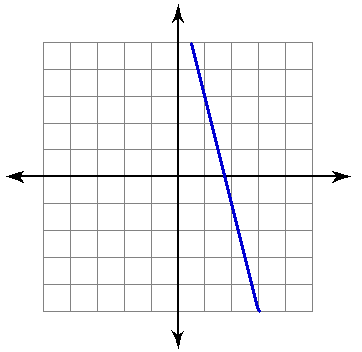8 Questions | Total Attempts: 401SettingsGiven two points, find the gradient of a line.

• 1.
Each grid line on the graph above is one unit. Find the gradient of the line in the graph above.
• 2.
Find the slope (gradient) that passes through the two points above. Give your answer as a fraction (ie. 3/4)
• 3.
Find the slope (gradient) that passes through the two points above. Give your answer as a fraction (ie. 3/4)
• 4.
Find the slope (gradient) that passes through the two points above. Give your answer as a fraction (ie. 3/4)
• 5.
Find the slope (gradient) that passes through the two points above. Give your answer as a fraction (ie. 3/4)
• 6.
Find the slope (gradient) that passes through the two points above. Give your answer as a fraction (ie. 3/4)
• 7.
Find the slope (gradient) that passes through the two points above. Give your answer as a fraction (ie. 3/4)
• 8.
Find the slope (gradient) that passes through the two points above. Give your answer as a fraction (ie. 3/4)
• 9.
Find the slope (gradient) that passes through the two points above. Give your answer as a fraction (ie. 3/4)
• 10.
Find the slope (gradient) that passes through the two points above. Give your answer as a fraction (ie. 3/4)
• 11.
Find the slope (gradient) that passes through the two points above. Give your answer as a fraction (ie. 3/4)
• 12.
Find the slope (gradient) that passes through the two points above.
• 13.
Find the slope (gradient) that passes through the two points above.
• 14.
Find the slope (gradient) that passes through the two points above.
• 15.
Find the slope (gradient) that passes through the two points above.
• 16.
Find the slope (gradient) that passes through the two points above.
• 17.
Find the slope (gradient) that passes through the two points above.
• 18.
Find the slope (gradient) that passes through the two points above.
• 19.
Find the slope (gradient) that passes through the two points above.
• 20.
Find the slope (gradient) that passes through the two points above.
• 21.
Find the slope (gradient) that passes through the two points above.
• 22.
Find the gradient of the line that passes through the two points above. Which of the choices below best describes the slope.
• A.

Positive

• B.

Negative

• C.

Zero

• D.

Undefined

• 23.
Find the gradient of the line that passes through the two points above. Which of the choices below best describes the slope.
• A.

Positive

• B.

Negative

• C.

Zero

• D.

Undefined

• 24.
Find the gradient of the line that passes through the two points above. Which of the choices below best describes the slope.
• A.

Positive

• B.

Negative

• C.

Zero

• D.

Undefined

• 25.
Find the gradient of the line that passes through the two points above. Which of the choices below best describes the slope.
• A.

Positive

• B.

Negative

• C.

Zero

• D.

Undefined

Related TopicsBack to top